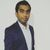# QlikView App Dev

Discussion Board for collaboration related to QlikView App Development.

Announcements
Join this live chat April 6, 10AM EST - QlikView to Qlik Sense REGISTER
cancel
Showing results for
Search instead for
Did you mean:Master

## Logic required

Hi guys

please help me in building below logic

I need last column

 A B C Result Cat 10 1 10 Tiger 54 2 10 Divide by 2 Dog 56 3 (10 Divide by 2)/3 Rabbit 34 4 ((10 Divide by 2)/3)/4 Pug Boss 43 5 (((10 Divide by 2)/3)/4)/5

Thanks

1 Solution

Accepted SolutionsMVP

May be this

=If(RowNo() = 1, B, Above(Result)/C)

or this

=If(RowNo() = 1, B, Above(Column(1))/C)4 RepliesCreator II

=(Result / Alt(above(Result),1))Master
Author

Not workingMVP

May be this

=If(RowNo() = 1, B, Above(Result)/C)

or this

=If(RowNo() = 1, B, Above(Column(1))/C)Master
Author

Thanks Sunny, got my mistake. small bracket played all the game